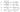# Selection Sort Algorithm

May 15, 2019It is one of a simple algorithm to study for a beginner to understanding sorting algorithms.

In this algorithm, we start with 0th index value. We compare it in whole array to find if any other number is smaller than this. So effectively, we find the minimum number through rest of the array from our starting index.

And, we swap the numbers of the initial number and the smalles number found in array.

## Selection Sort Algorithm

• Inner loop finds the minimum number
• Swap the indexes, if found the smaller number
• Inner loop breaks, Outer loop move ahead.
• So, we left the smallest number found so far in beginning. And, sort rest of the array

See the code here:

``````public void sort(int[] arr) {
int l = arr.length;
for (int i=0; i<l-1; i++) {
int smallestNumIndex = i;
for (int j=i+1; j<l; j++) {
if (arr[smallestNumIndex] > arr[j]) {
smallestNumIndex = j;
}
}
if (i != smallestNumIndex) {
//swap
int temp = arr[i];
arr[i] = arr[smallestNumIndex];
arr[smallestNumIndex] = temp;
}
}
}``````

## Graphical Example## Key Points

• Its an in-place sorting algorithm
• Performance is usually worse than Insertion sort
• Applicable for small set of input only
• Its very simple algorithm

## Runtime complexity

The algorithm runs on O(n^2) in worst/average case.